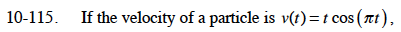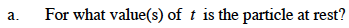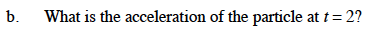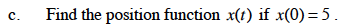### Home > CALC > Chapter 10 > Lesson 10.3.1 > Problem10-115

10-115.When t = 0 or cos(πt) = 0.a(t) = cos(πt) – t(π)sin(πt)$s(t)=\int v(t)dt$

Use integration by parts. Let f = f and dg = cos(πt)dt.

Solve for C given x(0) = 5.